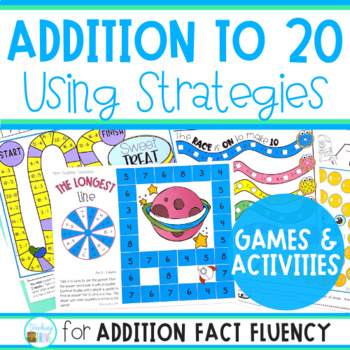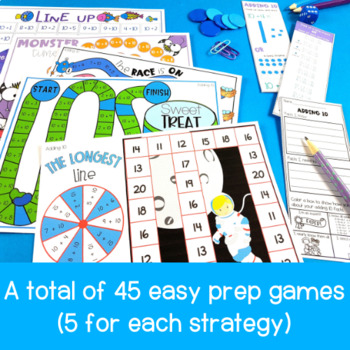# Addition Strategies for Number Fact FluencySubject
Resource Type
Format
PDF (32 MB|95 pages)
Standards
\$8.00
\$8.00

#### Also included in

1. Knowing and applying addition strategies and subtraction strategies help improve fact fluency. We all want our young learners to confidently answer their number facts with no hesitating or counting on fingers. To be able to fluently add or subtract within twenty using mental math strategies and know
\$12.50
\$16.00
Save \$3.50
2. Knowing and applying addition strategies and subtraction strategies help improve fact fluency. We all want our young learners to confidently answer their number facts with no hesitating or counting on fingers. To be able to fluently add or subtract within twenty using mental math strategies and know
\$22.00
\$32.00
Save \$10.00

### Description

Addition fact fluency is an important skill for all our young learners to develop. We want our students to confidently answer their addition facts with no hesitating or counting on fingers. To be able to fluently add within twenty using mental math strategies and know from memory all sums of two one-digit numbers is math standard for all second graders.

You can help them become fluent and increase their confidence in math by teaching them addition mental math strategies.

This math unit contains:

⇒ Teacher explanation for each strategy.

⇒ Anchor chart headings and number fact strips with instructions.

⇒ Interactive workbook foldable for using the above strategies. Students answer the number facts on the foldable.

⇒ Addition strategies placemat listing the addition mental math strategies and examples.

⇒ 5 colorful games (a total of 45 games) which can be used in pairs or small groups for consolidating each of the following strategies:

- Making 10

- Counting on 1, 2 and 3

- Doubles

- Near doubles (doubles + 1)

- Near doubles ( doubles + 2)

It's the perfect companion to Subtraction Strategies for Number Fact Fluency

For more practice with addition strategies try:

Addition Strategies - Games and Posters

Addition Strategies - Homework Games 2

Addition facts to 20 - Bump games

_______________________________________________________________________________

Become a follower and take advantage of my Early Bird Specials...all new products, excluding bundles, will be 50% off for the first 24 hours after being listed.

Total Pages
95 pages
N/A
Teaching Duration
N/A
Report this Resource to TpT
Reported resources will be reviewed by our team. Report this resource to let us know if this resource violates TpT’s content guidelines.

### Standards

to see state-specific standards (only available in the US).
Interpret whole-number quotients of whole numbers, e.g., interpret 56 ÷ 8 as the number of objects in each share when 56 objects are partitioned equally into 8 shares, or as a number of shares when 56 objects are partitioned into equal shares of 8 objects each. For example, describe a context in which a number of shares or a number of groups can be expressed as 56 ÷ 8.
Fluently add and subtract within 20 using mental strategies. By end of Grade 2, know from memory all sums of two one-digit numbers.
Add and subtract within 20, demonstrating fluency for addition and subtraction within 10. Use strategies such as counting on; making ten (e.g., 8 + 6 = 8 + 2 + 4 = 10 + 4 = 14); decomposing a number leading to a ten (e.g., 13 - 4 = 13 - 3 - 1 = 10 - 1 = 9); using the relationship between addition and subtraction (e.g., knowing that 8 + 4 = 12, one knows 12 - 8 = 4); and creating equivalent but easier or known sums (e.g., adding 6 + 7 by creating the known equivalent 6 + 6 + 1 = 12 + 1 = 13).
Relate counting to addition and subtraction (e.g., by counting on 2 to add 2).
Understand subtraction as an unknown-addend problem. For example, subtract 10 – 8 by finding the number that makes 10 when added to 8.